# 总结c++类的构造函数 拷贝构造函数 析构函数 赋值运算符重载的特点以及函数调用顺序

一 构造函数
1 构造函数： 构造函数时一个特殊的成员函数，用来初始化对象的数据成员，在对象创建时，由编译器自动调用，在对象的生命周期且只调用一次。
定义构造函数的原型的格式： 类名(形参列表)；
在类外定义构造函数的格式：类名::类名(形参列表)  {}

class Date        //date.h
{
public:
Date(int year, int month, int day);
private:
int _year;
int _month;
int _day;
};
Date::Date(int year,int month,int day)  //date.cpp
{
// 函数语句
}

2 构造函数的特点：

(1)函数名与类名相同；

(2)没有返回值，也不能指定为void类型

(3)有初始化列表

public:
Date(int year = 1995, int month = 12, int day = 8)
:_year(year)
,_month(month)
, _day(day)
{
}
初始化顺序：(1)每个成员在初始化列表中只能出现一次

(2)数据成员在类中定义的顺序就是参数列表中的初始化顺序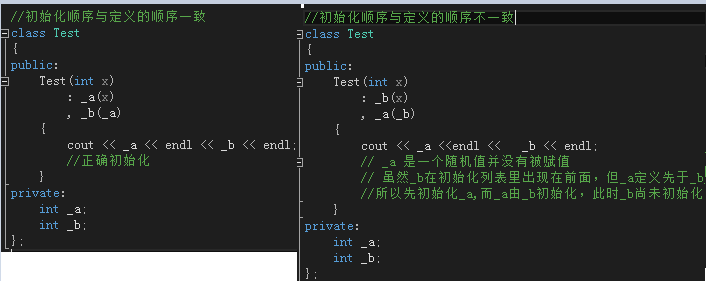类中以下成员必须放在初始化列表中初始化：

(a)引用数据成员 (b)  const 数据成员

(c)基类没有显示的构造函数，必须在派生类的构造函数中初始化基类构造函数

(d)类类型成员(该类没有缺省的构造函数)  因为初始化不必调用默认构造函数来初始化，直接调用拷贝构造函数

class Food
{
public:
Food(int q = 10)
{
_quantity = q;
cout << "food 构造" << endl;
}
Food(const Food& f)
{
_quantity = f._quantity;
cout << "food 拷贝构造" << endl;
}
private:
int _quantity;
};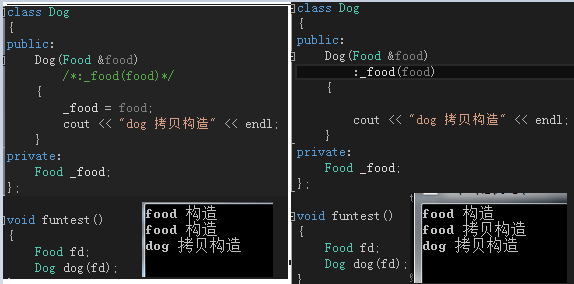（4）构造函数可以重载，默认参数只能在原型声明中指定，不能在函数定义中指定。

(5)无参构造函数和带缺省的构造函数都认为是缺省构造函数，并且缺省构造函数只能有一个

（6）构造函数不能用const 修饰

3 构造函数的作用 ：构建对象，初始化对象，类型转换

// 类型转换构造函数，根据一个指定的类型的对象创建一个本类的对象
// 例如：下面将根据一个double类型的对象创建了一个Complex对象
Complex::Complex(double r)
{
m_real = r;
m_imag = 0.0;
}

1  只有单个形参，而且该形参是对本类类型对象的引用（常用const修饰），创建对象时使用已存在的同类对象来进行初始化，由编译器自动调用

2 特征:(1)是构造函数的重载

(2)它的参数必须是同类类型对象的引用。原因：防止拷贝构造函数无限递归下去

(3)如果没有显示定义，系统会自动合成一个默认的拷贝构造函数。默认的拷贝构造函数会依次拷贝类的数据                   成员完成初始化。

3 使用场景：

class Date
{
public:
Date(int year = 1995,int month = 12,int day = 8)  // 构造函数
:_year(year)
, _month(month)
, _day(day)
{
cout << "date()构造函数"<< this << endl;
}
Date(const Date& d)//  拷贝构造函数
:_year(d._year)
, _month(d._month)
, _day(d._day)
{
cout << "date(&d)" << this << endl;
}
private:
int _year;
int _month;
int _day;
};

(1)对象实例化

Date t(1996,11,7);
Date t1(t);  // 调用拷贝构造函数
Date t2 = t; // 调用拷贝构造函数  此处先不考虑赋值操作符重载

(2)传值方式作为函数的参数

void Test(const Date d)  //传值方式作为函数的参数  调用拷贝构造函数
{
return;
}

(3)传值方式作为返回值

Date test()
{
Date date;  // 调用构造函数
return date;// 值传递作为函数返回值   调用拷贝构造函数
//return Date();  //  返回无名对象不掉用拷贝构造函数 只调用构造函数
}

Date& FunTest(Date& d)函数调用顺序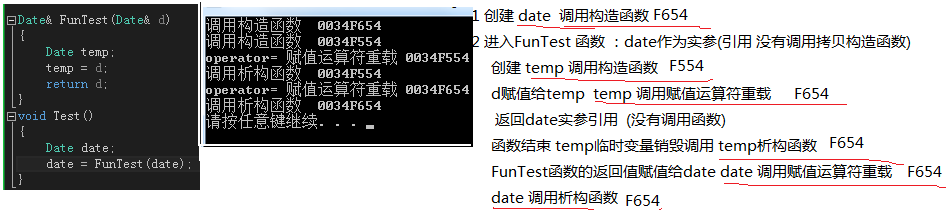Date FunTest(Date d)函数调用顺序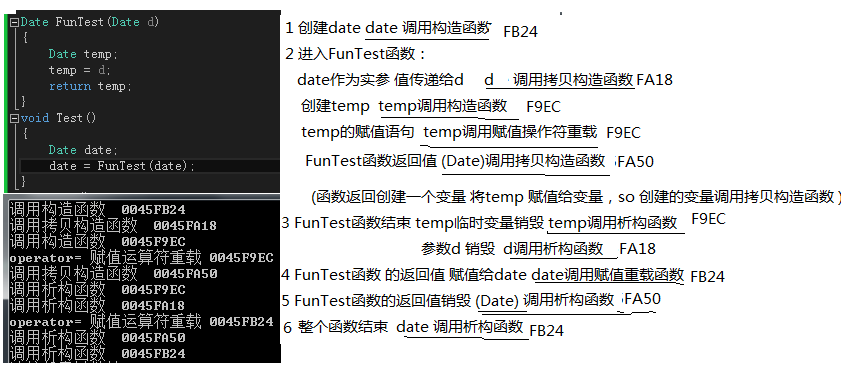Date FunTest(Date d)函数 返回值是无名对象调用顺序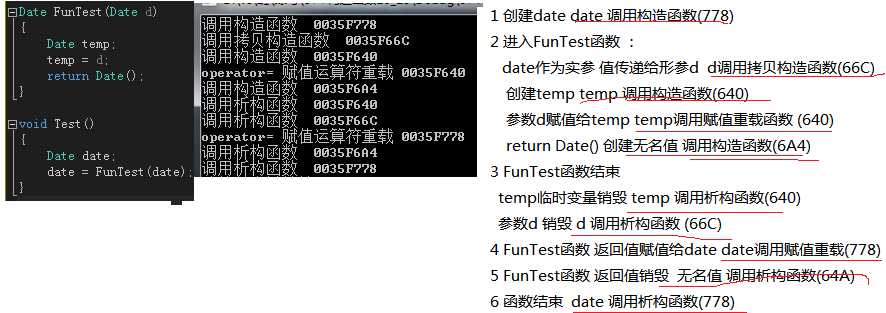<pre name="code" class="cpp">class A
{
…
private:
A(const A &a); // 私有的拷贝构造函数
};
A fun(A a){}

A  a;
A b(a);  // 错误，调用私有的拷贝构造函数
b = fun(a); // 错误 ，同上

析构函数:与构造函数的功能相反，在对象销毁时，由编译器自动调用，完成类的一些资源清理和汕尾工作。

class CArray
{
public:
CArray(size_t capacity) //构造函数
:_capacity(capacity)
{
_pData = (int *)malloc(capacity * sizeof(int));
_size = 0;
}
~CArray() //析构函数
{
if (NULL != _pData)
{
free(_pData);
_pData = NULL;
}
_size = 0;
_capacity = 0;
}
private:
int *_pData;
size_t _size;
size_t _capacity;
};

2 特性

a 析构函数没有参数没有返回值,函数名是类名前加上.

b 一个类有且只有一个析构函数。若未显示定义，系统会自动生成缺省的析构函数。

c析构函数并不是删除对象，只是做一些清理工作

d 调用次序与构造函数相反，最先构造的对象最后被析构。

c++中，对于任何一个类，如果没有用户自定义的赋值运算符函数，系统会自动的生成一个默认的(默认的完成的数据成员的逐位复制)。特殊情况下：如类中有指针形式就不能直接相互赋值，不然可能造成指针悬挂问题。

（2）赋值运算符重载函数不能被继承。

Date& Date::operator=(const Date& d) // 赋值运算符重载
{
cout << "operator= 赋值运算符重载 " << this << endl;
if (this != &d) // 判断是否自己对自己赋值
{
_year = d._year;
_month = d._month;
_day = d._day;
}
return *this;
}

void funTestOperator()
{
Date d1(2016, 10, 16);// d1 调用构造函数
Date d2(2016, 10, 17);// d2 调用构造函数
Date d3(2016, 10, 18); // d3调用构造函数
d1 = d2 = d3;  // 相当于d1.operator=(d2.operator=(d3))
// d2.operator=(d3) 调用一次赋值运算符函数，返回值1调用拷贝构造函数
// d1.operator=(返回值1) 调用第二次赋值运算符函数，返回值2调用拷贝构造函数
// 返回值2 调用析构函数 返回值d1 调用析构函数
}
// 函数结束调用d3 d2 d1 的析构函数

2 在赋值函数为：Date& operator=(const Date&d)的情况下

void funTestOperator()
{
Date d1(2016, 10, 16);// d1调用构造函数
Date d2(2016, 10, 17);// d2调用构造函数
Date d3(2016, 10, 18);// d3调用构造函数
d1 = d2 = d3; // 相当于d1.operator=(d2.operator=(d3))
// 调用两次赋值操作符函数
}

    Date d1,d3;
Date d2 = d1; // 拷贝构造函数
d3 = d1;  //赋值操作符重载函数`

04-02
03-1970
08-22719
04-15132
08-18443
08-0673
11-14151
09-291万+
05-308010

### “相关推荐”对你有帮助么？

•非常没帮助
•没帮助
•一般
•有帮助
•非常有帮助被折叠的  条评论 为什么被折叠?到【灌水乐园】发言¥2 ¥4 ¥6 ¥10 ¥20余额支付 (余额：-- )扫码支付获取中扫码支付点击重新获取扫码支付1.余额是钱包充值的虚拟货币，按照1:1的比例进行支付金额的抵扣。
2.余额无法直接购买下载，可以购买VIP、C币套餐、付费专栏及课程。余额充值